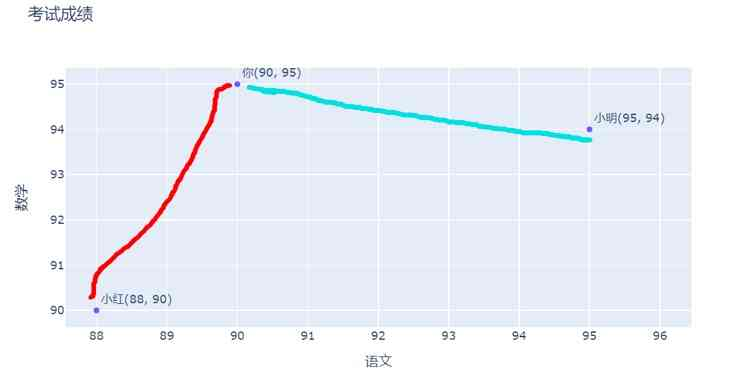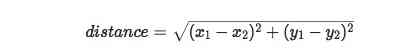# 用python从零开始写一个推荐系统(1)

4.5 3.4 4 4.8

1. 欧几里得距离
2. 皮尔逊相关度

## 2. 欧几里得距离

90 95

``````import plotly
import plotly.offline as py
py.init_notebook_mode(connected=False)
import plotly.graph_objs as go

dataset = {
'语文': [90, 95, 88],
'数学': [95, 94, 90],
}

data_g = []

data = go.Scatter(
x=dataset['语文'],
y=dataset['数学'],
text = ['你(90, 95)', '小明(95, 94)', '小红(88, 90)'],
mode='markers+text',
textposition="top right",
name='考试成绩')

data_g.append(data)

layout = go.Layout(title="考试成绩",
xaxis={'title': '语文'}, yaxis={'title': '数学'})
fig = go.Figure(data=data_g, layout=layout)
fig.show()
````````````import math

distance = math.sqrt(pow(90 - 88, 2) + pow(95 - 90, 2))
print(distance)     # 5.385164807134504
``````

``````import math

distance = math.sqrt(pow(90 - 95, 2) + pow(95 - 94, 2))
print(distance)     # 5.0990195135927845
``````

90 95 98

``````import math

# 你和小明的距离
distance1 = math.sqrt(pow(90 - 95, 2) + pow(95 - 94, 2) + pow(98 - 94, 2))
print(distance1)     # 6.48074069840786

# 你和小红的距离
distance1 = math.sqrt(pow(90 - 88, 2) + pow(95 - 90, 2) + pow(98 - 97, 2))
print(distance1)     # 5.477225575051661
``````

``````1/(1+distance)
``````

``````def sim_distance(lst1, lst2):
sum_value = 0
for x1, x2 in zip(lst1, lst2):
sum_value += pow(x1 - x2, 2)
return 1/(1 + math.sqrt(sum_value))
``````

## 3. 皮尔逊相关系数

90 95 98

1. 分数上看，你的英语分 > 数学分 > 语文分， 小刚也呈现出相同的规律
2. 小刚各科的分数都比你少5分，你们的数学都比语文多5分，英语都比数学多3分
3. 反观小明，他语文成绩比你好，数学和英语分数相同，而你的英语比数学好，他的各科分数 语文分 > 数学分 = 英语分

``````import math
import numpy as np

lst1 = [90, 95, 98]
lst2 = [95, 94, 94]
lst3 = [85, 90, 93]

def cal_pccs(x, y):
'''
皮尔逊相关系数
'''
count = len(x)
sum_xy = np.sum(np.sum(x*y))
sum_x = np.sum(np.sum(x))
sum_y = np.sum(np.sum(y))
sum_x2 = np.sum(np.sum(x*x))
sum_y2 = np.sum(np.sum(y*y))
pcc = (count*sum_xy-sum_x*sum_y)/np.sqrt((count*sum_x2-sum_x*sum_x)*(count*sum_y2-sum_y*sum_y))
return pcc

def sim_distance(lst1, lst2):
sum_value = 0
for x1, x2 in zip(lst1, lst2):
sum_value += pow(x1 - x2, 2)
return 1/(1 + math.sqrt(sum_value))

print(cal_pccs(np.array(lst1), np.array(lst3)))     # 1.0
print(sim_distance(lst1, lst3))     # 0.10351694645735657

print(cal_pccs(np.array(lst1), np.array(lst2)))     # -0.9285714285714286
print(sim_distance(lst1, lst2))     # 0.13367660240019172
``````QQ交流群: 211426309